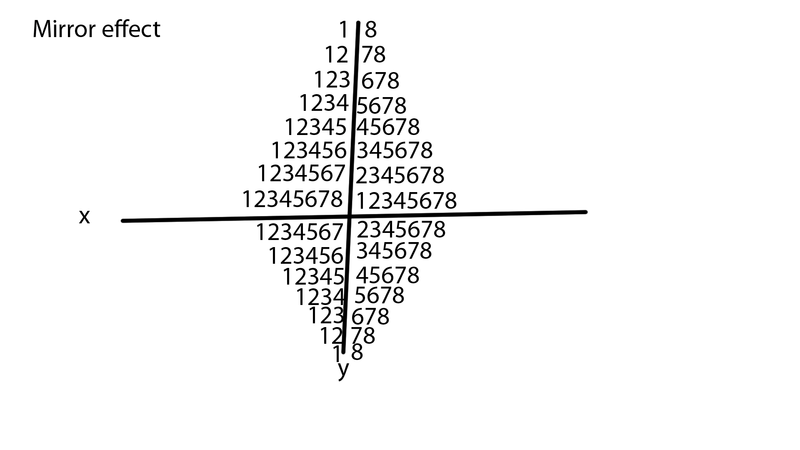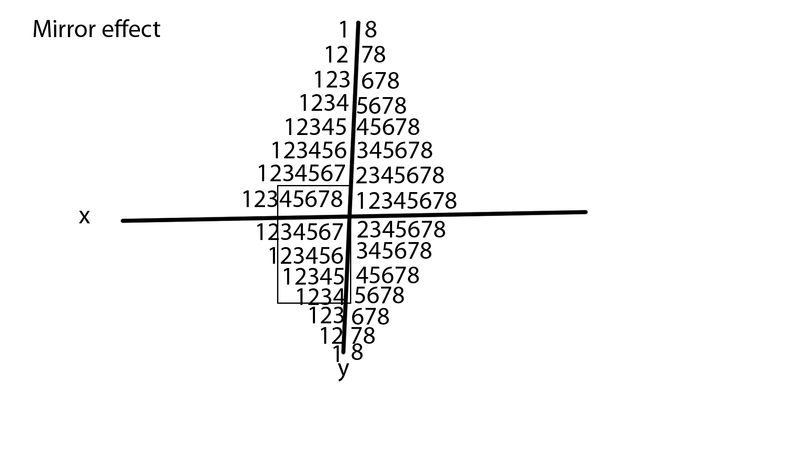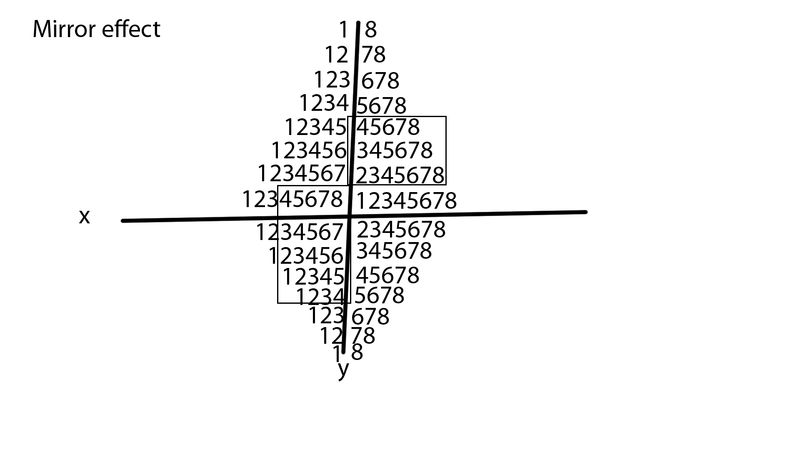User Name Remember Me? Password

 Math General Math Forum - For general math related discussion and news

 January 9th, 2017, 10:48 AM #1 Newbie   Joined: Jan 2017 From: ohio Posts: 2 Thanks: 0 Math without zero? Hey guys I need help with something. Basically I was thinking about doing math without the number 0, I figured using only the digits 123456789 I could arrange them to create the pattern you see here:If you take notice, all numbers corresponding to each other = 9 (1+8=9 )(2+7=9) (3+6=9)(4+5=9) then reverse (5+4=9)(6+3=9)(7+2=9)(8+1=9) So I thought what if I make a graph using this idea, Here is what I came up with:If you will notice, all I simply did was write 12345678 and then in then next column I again wrote 12345678 in order to do this again I simply could have started with the 2 and wrote 2345678 going down(vertical). Instead, I wrote them going left to right (horizontal). As you can see the numbers split apart but always combine to 9 which they would have done no matter how I decided to write them. Now you may ask "but what is so special about 9" well as it so happens every equal degree is 9 as well (18,27,36,45,54,63,72,81,90,180,270,360 etc.) Also, you might be interested to know that our numbers represent angles (90 degree angles) So basically you are writing 1(90) 2(180) 3(360) 4(450) 5(540) 6(630) 7(720) 8(810) 9(900) radian 9: 0.15708(1+5+7+0+8 = 21(3) radian 90: 1.+5+7+0+8 = 21(3) radian 18: 0.314159 =23(5) radian 180: 3.14159(5) radian 27: (0.4+7+1+2+3+9 =26(8) radian 270: 4+.7+1+2+3+9(8) radian 36: 0.6+2+8+3+1+9 = 29(1) radian 360: 6+.2+8+3+1+9 = 29(1) radian 45: 0.7+8+5+3+9+8 = 40(4) radian 450 7.85398 = 40(4) radian 54: 0.9+4+2+4+7+8 = 34(7) radian 63: 1+.0+9+9+5+6 = 30(3) radian 72: 1+.2+5+6+6+4 = 24(6) radian 81: 1.+4+1+3+7+2 = 18(9) radian 90: 1.+5+7+0+8 = 21(3) radian 180: 3.14159(5) radian 270: 4+.7+1+2+3+9(8) radian 360: 6+.2+8+3+1+9 = 29(1) radian 450 7.85398 = 40(4) Here amazingly (at least in my mind) you can see that when you calculate the radians in such a manner every number appears except for 2. I was also surprised to see the first 6 digits for pi moved one decimal place to the right when I looked at angle 18 and then they aligned with 180 Note: I wanted to stop calculating with 0s when I seen the pattern appearing to be the same, So I did, you can check the rest if I have peaked your interests. Also this is primarily research based on geometric patterns in nature and my understanding of a "paradox" Basically my understanding of a paradox is 2 mirrors placed on either side and viewing it from the center. The idea is that everything mimics everything in a parallel manor. More easily put, put your hands together and open them equally, You will see the same thing happening except your hands will mirror one another. Questions: Where is the number 2 in the calculations for radians? What could 0s and .(decimal points) mean? Do I need to include Z axis in order to calculate this? Last edited by skipjack; January 9th, 2017 at 01:12 PM.January 9th, 2017, 01:02 PM #2 Senior Member   Joined: Feb 2016 From: Australia Posts: 1,834 Thanks: 650 Math Focus: Yet to find out. The Romans seemed to manage.. For their time.January 9th, 2017, 01:37 PM   #3
Global Moderator

Joined: Dec 2006

Posts: 20,929
Thanks: 2205

Quote:
 Originally Posted by Michaelsrk. . . the pattern you see here:
You posted a non-working link, but probably intended the diagram below.
NinePointCircle.JPGJanuary 9th, 2017, 05:25 PM #4 Newbie   Joined: Jan 2017 From: ohio Posts: 2 Thanks: 0 I'm thinking that 0 is the line dividing 8 from 1 soorJanuary 17th, 2017, 09:15 AM #5 Global Moderator   Joined: Dec 2006 Posts: 20,929 Thanks: 2205 Did you have any particular reason for avoiding use of zero?January 17th, 2017, 09:40 AM   #6
Math Team

Joined: Dec 2013
From: Colombia

Posts: 7,674
Thanks: 2654

Math Focus: Mainly analysis and algebra
Quote:
 Originally Posted by MichaelsrkAlso, you might be interested to know that our numbers represent angles (90 degree angles) So basically you are writing 1(90) 2(180) 3(360) 4(450) 5(540) 6(630) 7(720) 8(810) 9(900)
This is complete nonsense. Arabic numerals are derived from ancient indian script. Not all the shapes are preserved down the ages and "angles" certainly aren't.January 17th, 2017, 08:46 PM   #7
Senior Member

Joined: Sep 2016
From: USA

Posts: 635
Thanks: 401

Math Focus: Dynamical systems, analytic function theory, numerics
Quote:
 Originally Posted by v8archieThis is complete nonsense. Arabic numerals are derived from ancient indian script. Not all the shapes are preserved down the ages and "angles" certainly aren't.
This is a huge pet peeve of mine. Its probably not rational but I absolutely hate people who make up bullshit like this and even go so far as to make an entire video of this stupidity. Glad someone called it out.Tags mathSearch tags for this page

### math without zero

Click on a term to search for related topics.
 Thread ToolsShow Printable VersionEmail this Page Display ModesLinear ModeSwitch to Hybrid ModeSwitch to Threaded ModeSimilar Threads Thread Thread Starter Forum Replies Last Post snezana Math Software 0 March 1st, 2016 12:28 AM v4formath Math Books 0 August 15th, 2014 07:04 PM Sid Math Books 5 May 27th, 2014 12:20 PM chuckycheese Elementary Math 1 May 22nd, 2014 03:45 AM Sid Academic Guidance 2 May 11th, 2014 07:23 AM

 Contact - Home - Forums - Cryptocurrency Forum - Top

Copyright © 2019 My Math Forum. All rights reserved.